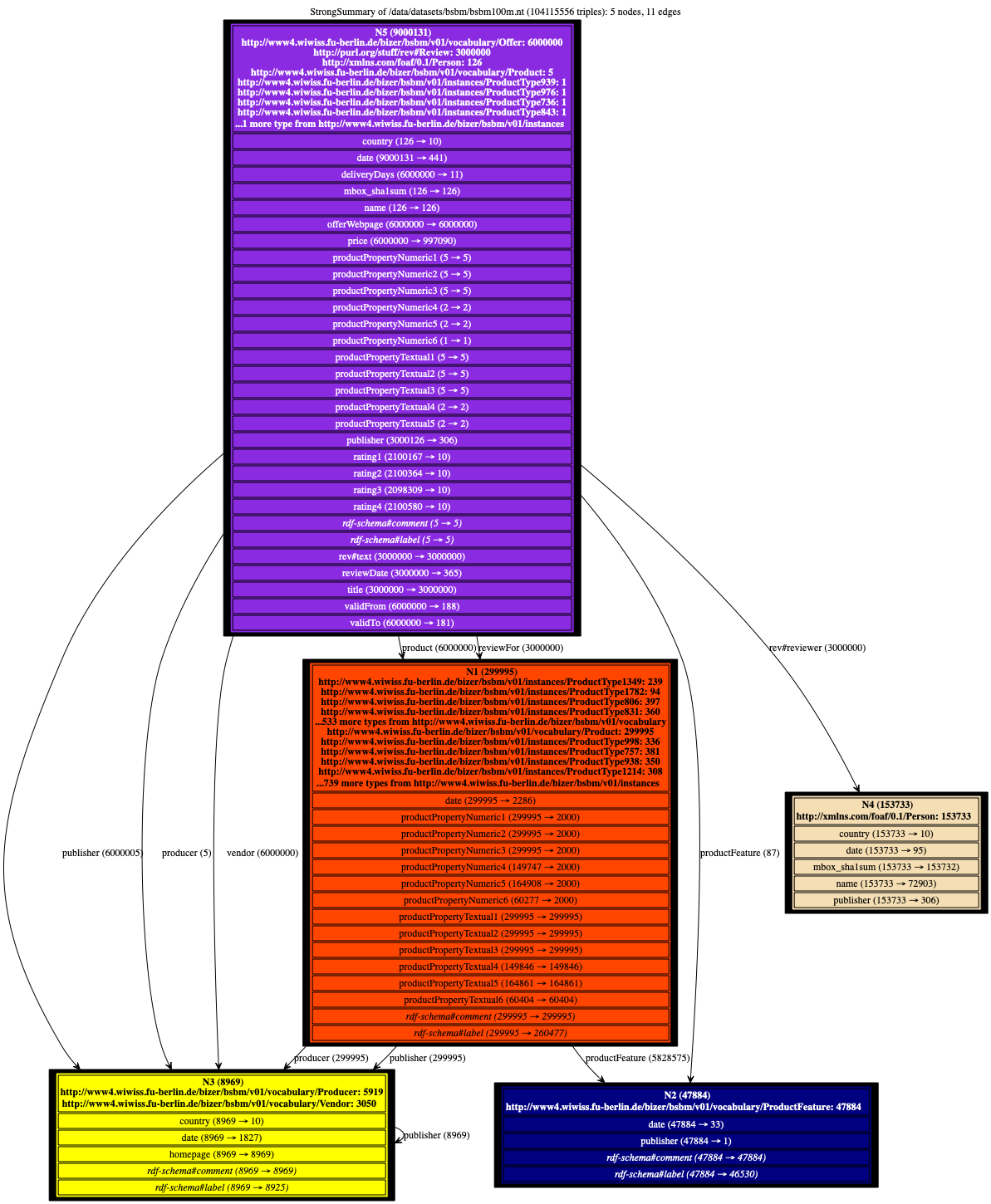# How to read a visualization

### How to read a visualization?Each summary node in such a visualization reads as follows:

• A certain number of nodes from the RDF graph are represented by the summary node, e.g., N5 represents 9.000.131 RDF nodes in the above graph;
• Some of these nodes have some of the types represented in the top of the node box, the precise counts are shown under the node name. For instance, 6.000.000 of the nodes represented by N5 are of type Offer, while 3.000.000 are of type Review;
• Together, the nodes represented by N5 have a certain number of outgoing properties which lead to leaf nodes. For instance, there are 126 country edges outgoing these nodes, which lead to 10 distinct nodes; there are 9.000.031 date edges, leading to a total of 441 distinct dates etc.
• Together, the nodes represented by N5 have a certain number of outgoing properties which lead to non-leaf nodes. For instance, there are 6.000.000 product edges leading from node(s) represented by N5, to some node(s) represented by N1.
• Some nodes have some generic properties which were not taken into account to determine which nodes are equivalent. This is the case for instance of the rdfs#label properties of the nodes represented by N5. Generic properties are shown in italic.